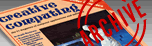`CREATIVE COMPUTING VOL. 11, NO. 4 / APRIL 1985 / PAGE 98`

 More than one way to skin a rabbit; the Fibonacci sequence revisited. David H. Ahl. Many moons ago, we printed a letter from Konrad Kossman with a longish program for producing the Fibonacci sequence. In his letter he asked if there was a better way. I offered two alternatives, admittedly written in some haste. Readers replied with a vengeneance pointing out several additional way to generate this famous sequence. But before listing some of these gems, let us put the problem in a somewhat better historical perspective. Leonardo Fibonacci was an Italian mathematician who lived in the late 12th and early 13th centuries. In Liber abaci (1202), for centuries a standard work on arithmetic and algebra, he advocated the adoption of Arabic notation. In Practica geometriae (1220) he organized and extended the material then known in geometry and trigonometry. In Liber abaci, Fibonacci proposes an interesting problem of the rabbits. Suppose we put a pair of adult breeding rabbits in a cage to produce offspring and that after two months and each month thereafter they produce another pair, which, in turn, breed after two months. (This is hypothetical, of course, as rabbits do not reach maturity before four months of age.) If all the rabbits survive, how many pairs will there be at the end of any given month, or the end of one year? The solution to the problem can be easily diagrammed--at least for the first six months or so. If you draw a rabbit diagram, you will find the number of pairs of rabbits in successive months is 1, 1, 2, 3, 5, 8, 13, 21... Fibonacci did not explore the question of sequences more deeply, and it was not until the 19th century that Francois Edouard Anatole Lucas investigated the Fibonacci series and formally stated that each term is the sum of the two before. Thus, it invites computation by means of a computer program. In the original article, my little three-line program to generate the series was as follows: 10 X=1:Y=1:PRINT 1;1; 20 Z=X+Y:PRINT Z; 30 X=Y:Y=Z:GOTO 20 Several readers pointed out that it is unnecessary to print out the first two elements of the series. Martin Mersky of Phoenixville, PA showed that the program could be easily amended to generate the entire sequence. 10 X=1 20 Z=X+Y:PRINT Z; 30 X=Y:Y=Z:GOTO 20 A further modification which Martin feels illustrates what is actually going on, and is also simple elegant is as follows: 10 X=1 20 Y=X:X=Z 30 Z=X+Y:PRINT Z;:GOTO 20 Ramunas Motekaitis of College Station, TX commented that my program disturbed his sixth sense of efficient programming and asked, rhetorically, "Why code more than is absolutely necessary?" Here is his program: 10 Y=1 20 PRINT Y;:Z=X+Y 30 X=Y:Y=Z:GOTO 20 Mikko Nieminen of Finland took a somewhat different approach which calculates two new terms in each loop. Not surprisingly, all five of these programs run at exactly the speed. 10 X=1:Y=1 10 PRINT X;Y; 30 X=X+Y:Y=Y+Y:GOTO 20 All of the above approaches to calculating the Fibonacci sequence use recursion and must calculate every number in the sequence to reach a given point. However, George Miller of San Francisco and Joseph Freedman of Willow Grove, PA both mentioned a wonderful formula from linear algebra that produces any given number in the series. Fn = (1/[square roots]5) [((1 + =square roots])/2).sup.n - ((1 - [square roots]5)/2).sup.n] This formula is discussed in detail in Donald E. Knuth's book, The Art of Computer Programming, Vol. 1 (pp. 78-83). Simply stated, the closed form of a function is different from the iterative and the recursive forms in that you furnish a number, n, and the formula calculates the value for that number. You can easily write a program with the above formula to calculate Fibonacci numbers, but it will not work! The reason is that the computer uses an approximation to calculate the value of the square root of 5. Here are two programs that use this formula to calculate any Fibonacci number, the first by George Miller and the second by Joseph Freedman. Notice the corrections for the roundoff errors. 10 FOR N=1 TO 20 20 PRINT INT ((((1+SQR(5))/2)*N-((1-SQR(5))/2)*N)/SQR(5)+.5); 30 NEXT N To generate an entire sequence of numbers, the formula method is considerably slower than the recursive approach; however, to generate just one number, particularly a high order one, the formula is certainly preferred. Miller's program, incidentally, is somewhat faster than Freedman's. To Mr. Fibonacci go our thanks for his rabbit problem; and to our readers, thanks for helping skin it in a variety of interesting ways!